# Building toward fluency

Alignments to Content Standards: 2.OA.B.2

#### Materials

• Whiteboard or chart paper and markers
• Empty number line or magnetic cubes lined up on the whiteboard, alternating colors every 5 (see solution)
• List of expressions ready to write up on the board:
1. 4+10
2. 4+12
3. 4+22
4. 8+20
5. 8+29

#### Actions

• Write the expression on the board or chart paper. Start with 4+10.
• Ask students to describe their strategy for solving the problem.
• Choose one or more students to explain their strategy to the class. Represent each strategy on the board using the number line or magnetic cubes (see solution).
• Once the student's strategy is understood by the class, continue with the next sum.

## IM Commentary

The purpose of this task is to promote certain addition strategies that will help students learn to fluently add and subtract within 20. "Computational fluency refers to having efficient, accurate, generalizable methods (algorithms) for computing numbers that are based on well-understood properties and number relationships" (NCTM, 2000). Therefore, the focus in developing fluency should be more than the internalization of facts but on supporting students natural development of number sense so that they are able to solve computations flexibly and efficiently using their understanding of place value and relationships between numbers.

The pairs of numbers students are asked to add are carefully chosen to help students develop efficient and flexible computational skills and to develop a variety of mental math strategies for computational fluency. The first problem is a scaffold to the second. Assuming that children know the pattern of adding 10, this set encourages them to build from this understanding. The second expression has a value just 2 more than the first, and students may see the connection between the first and second problem and adding the two units. The third expression has a value 10 greater than the second and the initial representation of tens are used – but only if students offer this strategy. The next two problems are paired, since 29 is 9 more than 20. This problem also provides the opportunity to think of 29 as 30-1.

Using an open number line or a number line created by a train of cubes (see solution section below for example) will help students visualize the relationship between numbers. Pure verbal explanations can sometimes be difficult for children (and even adults) to understand. Having a visual representation allows more students to understand, take part in the discussion, and make use of the representation for further problems.

Children’s natural development of numbers progress from the concrete to the abstract, from counting all (e.g. physically making four counters and then making twelve and counting all the counters to get sixteen), to counting on (e.g., counting four more starting at twelve to get to sixteen), to using part-whole (e.g. splitting apart the twelve to ten and two, and adding the two to four, then adding the ten) and relational thinking (knowing that 4 + 10 is 14 so 4 + 12 would be just two more).

This task is based on Cathy Fosnot’s work using number strings – a string of related computational problems that are strategically chosen to connect specific concepts of numeracy. For more information and video demonstrations on addition and subtraction strings: http://mathcoachondemand.blogspot.com/2011/03/mental-math-strings.html http://erlc.ca/resources/resources/math_vodcasts/number_strings_to_encourage_addition_strategies_part2.php http://www.contextsforlearning.com/gradesK_3/unitBooksAnnotated.asp

Reference

National Council of Teachers of Mathematics. (2000). Principles and standards for school mathematics. Reston, VA: Author.

## Solution

1. 4 + 10 is 14. 10 more than 4 is 14. Here is how it would look using the magnetic cubes:Here is how it would look on an empty number line: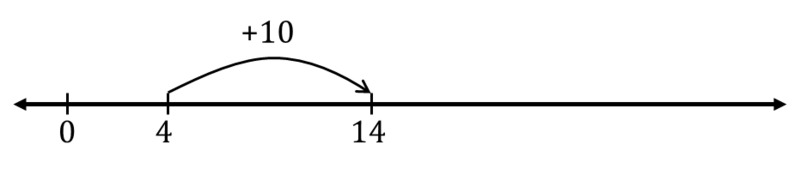2. 4 + 12 is 16 because it is two more than the last problem. Here is how it would look using the magnetic cubes: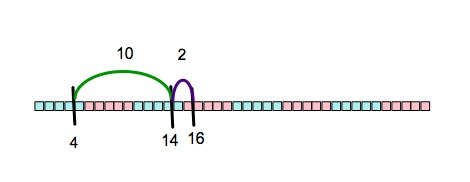Here is how it would look on an empty number line:3. 4+22 is 26. 2 and 4 is 6 and 10+ 10 + 6.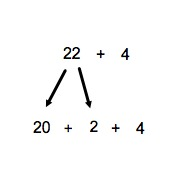We can also use the last problem. Just add on 10 more to 4+12: so 16, then 26.Here is how it would look using the magnetic cubes:Here is how it would look on an empty number line: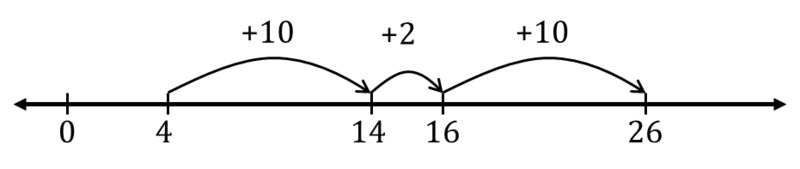4. 8 + 20 is 28. 10 more than 8 is 18, and 10 more than 18 is 28. Here is how it would look using the magnetic cubes:A student may also use the commutative property, 20 + 8 = 28. 8 more than 20 is 28. Here is how it would look using the magnetic cubes.5. 8 + 29 = 37. 8 + 9 is 17, and 20 + 17 is 37.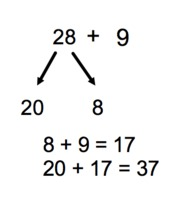A student may use the last problem. Just add on 10 more to 28; so 38, and take away 1 to get 37. Here is how it would look using the magnetic cubes: